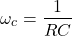# Cutoff Frequency: What is it? Formula And How To Find it

Contents

## What is Cutoff Frequency?

Cutoff frequency (also known as corner frequency, or break frequency) is defined as a boundary in a system’s frequency response at which energy flowing through the system begins to be attenuated (reflected or reduced) rather than passing through.

The cutoff frequency or corner frequency in electronics is the frequency either above or below which the power output of a circuit, such as a line, amplifier, or electronic filter (e.g. a high pass filter) has fallen to a given proportion of the power in the passband.

Most frequently this proportion is one-half the passband power, also referred to as the 3 dB point since a fall of 3 dB corresponds approximately to half power. As a voltage ratio, this is a fall to approximately 0.707.

For any filtering circuits such as RC circuits, the cutoff frequency is a very important characteristic. At this point, the amount of attenuation due to the filter starts to increase swiftly.

To indicate how long the amplifier gain can remain constant in frequency, we need to define a range of frequencies. Along with that range, the gain should not deviate more than 70.7% of the maximum gain that has been defined as a reference at mid-frequency. In the below-shown curve, f1 and f2 indicate lower cut-off and upper cut-off frequencies.

## What is Bandwidth?

In signal processing, bandwidth is defined as the difference between the upper cutoff frequency and low cutoff frequency. The frequency f2 lies along with a high-frequency range and f1 in the low-frequency range. We can also name these two frequencies as Half–Power frequencies since voltage gain drops to 70.7 % of the maximum value.

This represents the power level of one–half of the power at the reference frequency in the mid-range frequency. Since the change is not noticeable, the audio amplifier has a flat response from f1 to f2.

## Cutoff Frequency Formula

The formula for cutoff frequency (corner frequency) is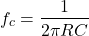where R and C are the values of Resistance and Capacitance. For a simple RC low pass filter, cut-off (3dB point) is defined as when the resistance is the same magnitude as the capacitive reactance

## Decibel Unit

Gain is usually expressed in decibels. The decibel unit arises from the logarithmic response of the human ear to the intensity of sound. Hence decibel is given as a logarithmic measurement of the ratio of one power to another, it can also be expressed as a ratio of one voltage to another.

Generally, the voltage output or voltage gain of an amplifier is expressed in decibels (dB) which is given by Voltage gain in dB is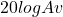.

The power gain of the amplifier is expressed in decibels (dB) which is given by power gain in dB is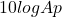When Av is greater than one, it is said that dB gain is positive. It represents the amplification. When Av is less than one, dB is negative. It represents attenuation.

In amplifiers under a few circumstances, the value of gain may be assigned with a 0 dB reference. In such situation, it means that reference gain is used as a reference which is used to compare other values of gain.

Amplifiers show a maximum gain in the mid-frequency range and reduced gain in the low-frequency range. Maximum gain is called the mid-frequency range with a value of 0 dB. When the gain value is below the mid-frequency range, it is expressed as a negative dB value.

## How to Find Cutoff Frequency

There are many methods by which cutoff frequency can be calculated.

### Cutoff Frequency from Transfer Function

Analysis of a circuit with an altering frequency of sinusoidal sources is termed the frequency response of a circuit. The transfer function of a circuit is defined as the ratio of the output voltage to the input voltage in the s domain.When using a sinusoidal source, the transfer function will be given as the magnitude and phase of the output voltage to the magnitude and phase of the input voltage in a circuit. In such a case,will be used instead of s.For example, consider the transfer function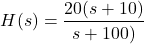To obtain the corner frequency from the above equation, H(s) can be replaced as

(1)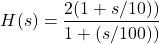(2)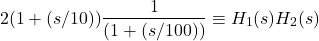So, from this equation, the corner frequency is calculated asand. In order to choose the range of frequencies, we must consider the values of corner frequency.

### Cutoff Frequency from Bode Plot

A graph that is commonly used in control system engineering to determine the stability of a control system is known as a Bode plot. The Bode plot outlines the frequency response of the system by two graphs – the Bode magnitude plot (which shows the magnitude in decibels) and the Bode phase plot (which shows the phase shift in degrees).

In the Bode plot, the corner frequency is defined as the frequency at which the two asymptotes meet each other or cut each other.

The transfer function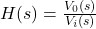of a system carries extensive information about the gain and stability of the system. Bode plots give an estimated picture of a given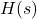from which a fair idea of the gain of the system and its stability properties can be
achieved.

### Cutoff Frequency of a Low Pass Filter

A low-pass filter is a circuit that allows low-frequency signals and stops high-frequency signals. All low-pass filters have a certain cutoff frequency, above which the output voltage drops below 70.7% of its input voltage. The frequency at which the magnitude response is 3 dB lower than the value at 0 Hz, is known as the Cutoff Frequency of a low pass filter.

For example, if a low pass capacitive filter has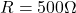and, at what frequency will the output be 70.7%?

A simple capacitive low pass filter with one resistor and one capacitor has a cutoff frequency of. Substituting the corresponding R and C values, the cut-off frequency would be 45.473 Hz. So, the output will be 70.7% at 45.473 Hz.

When Bode Plot is plotted for a low pass filter as shown in the image below, the frequency response of the filter seems to be nearly flat for low frequencies.

Until the Cut-off Frequency point is reached, all of the input signals pass directly to the output, which results in a unity gain. This happens when the reactance of the capacitor is large at low frequencies and prevents any current flow through the capacitor. The response of the circuit decreases to zero at a slope of -20dB/ Decade “roll-off” after this cut-off frequency point.

The frequency point at which the capacitive reactance and resistance are equal is known as the cutoff frequency of a low-pass filter. At cutoff frequency, the output signal is attenuated to 70.7% of the input signal value or -3dB of the input.

Consider a first-order low pass filter with a transfer functionRephrase the above equation by dividing the numerator and denominator by RC

(3)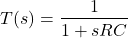(4)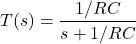Hence,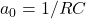and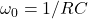, where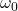is the cutoff frequency.

To have a better understanding of cut-off frequency, convert the standard s domain transfer function into equivalentformat.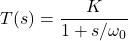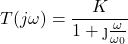Now, lets evaluate this expression at cutoff frequency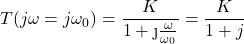The denominator being a complex number, the magnitude has to be calculated.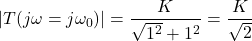K is the DC gain. When the input frequency increases to the cut-off frequency, the output amplitude will be. The value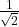corresponds to -3dB which is nothing but the cutoff frequency.

This transfer-function analysis has shown clearly that the cutoff frequency is just the frequency at which the filter’s amplitude response is decreased by 3 dB corresponding to the very-low-frequency amplitude response.

### Cutoff Frequency of a High Pass Filter

A high-pass filter passes signals with a frequency greater than a specified cutoff frequency. It attenuates signals with frequencies lower than that cutoff frequency.

The transfer function is derived in the below equations.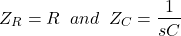The output impedance is given as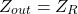Input impedance is given as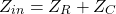The transfer function of a high pass filter is defined as the ratio of Output voltage to the input voltage.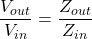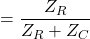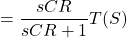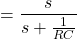On comparing the above equation, with the standard form of the transfer function,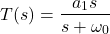is the amplitude of the signal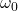is the angular cutoff frequency

The cutoff frequency is known as a frequency creating a boundary between the pass and stop band. If the signal frequency is more than the cutoff frequency for a high pass filter then it will cause the signal to pass. The cutoff frequency equation for the first-order high pass filter is the same as the low pass filter.### Cutoff Frequency of a Bandpass Filter

The Band Pass Filter consists of two cutoff frequencies. The bandpass filter is made of a high pass and a low pass filter. The first cutoff frequency is from a high pass filter, known as the higher cutoff frequency. This cut-off frequency is known as FC high.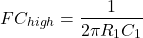The second cutoff frequency is from the low pass filter known as the lower cutoff frequency. This cut-off frequency is known as FC low.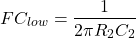Bandwidth is given as the range between these frequencies. For a high pass filter, the cut-off frequency will define the lower value of bandwidth. For a low pass filter, the cutoff frequency will define the higher value of bandwidth.

### Cut off Frequency of RL circuit

Consider a simple RL circuit as shown below.

The transfer function for the same is given as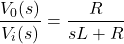Substitute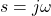in the above equation to calculate the frequency response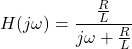Magnitude Response is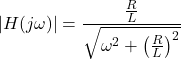When= 0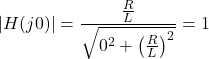When=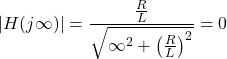To calculate the cutoff frequency,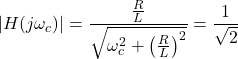Finally, cut off frequency of an RL circuit is given as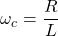### Cut off Frequency of RC circuit

Consider a simple RC circuit as shown below.

The transfer function for the same is given as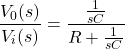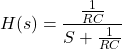Substitutein the above equation to calculate the frequency response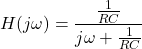Magnitude Response isWhen= 0When=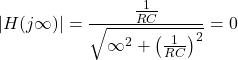To calculate the cutoff frequency,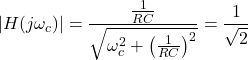Finally, cut off frequency of an RL circuit is given as